# Setting and Sedimentation (Part - 1) Computer Science Engineering (CSE) Notes | EduRev

## Computer Science Engineering (CSE) : Setting and Sedimentation (Part - 1) Computer Science Engineering (CSE) Notes | EduRev

The document Setting and Sedimentation (Part - 1) Computer Science Engineering (CSE) Notes | EduRev is a part of the Computer Science Engineering (CSE) Course Environmental Engineering - Notes, Videos, MCQs & PPTs.
All you need of Computer Science Engineering (CSE) at this link: Computer Science Engineering (CSE)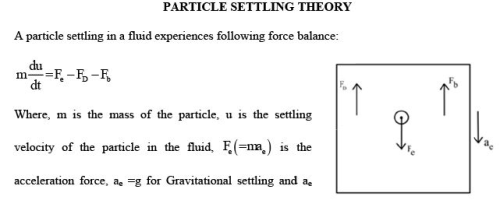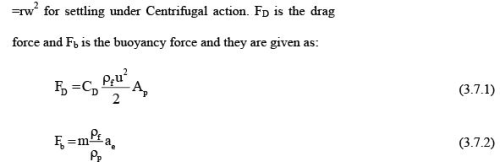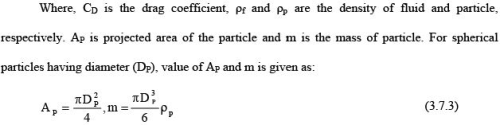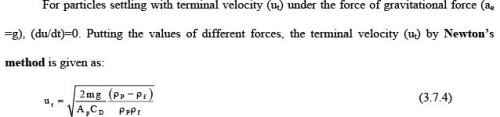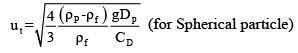(3.7.5)

Variation of CD (Drag-coefficient)

In laminar zone, Stoke’s law is applicable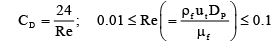(3.7.6)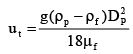(3.7.7)

For transition zone,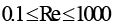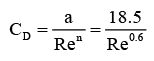(3.7.8)

For turbulent zone, CD is independent of Re and CD=0.4

For non-spherical particles, formula for Reynold number and settling velocity calculation are modified using the shape factor ( φ ) :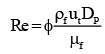(3.7.9)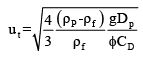(3.7.10)

Problem 3.7.1: A sand particle has an average diameter of 1 mm and a shape factor of 0.90 and a specific gravity of 2.1, determine the terminal velocity of the particle settling in water at 20 oC (kinematic viscosity of water=1.003×10-6 m2/s and specific gravity=1). Drag coefficient can be computed using the following equation: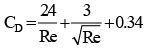Solution: kinematic viscosity(V) =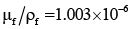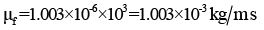Settling velocity using stokes law is: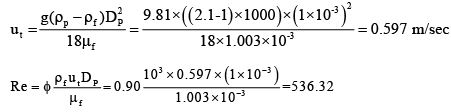Since Re>1, therefore, Newton’s law should be used for finding terminal velocity in transition zone. For initial assumption of settling velocity, stoke’s law is used. This initially assumed velocity is used to determine the Reynold number which is further used to find settling velocity. This iterative procedure is repeated till initial assumed velocity is approximately equal to settling velocity calculated from Newton’s equation.

Initial drag coefficient is calculated as: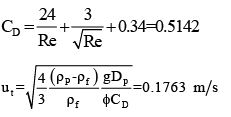Now, iterative procedure is continued: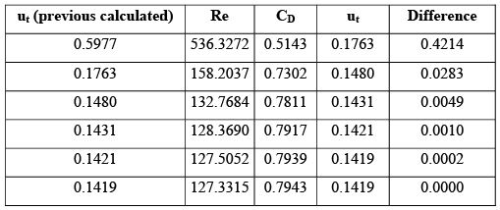Final settling velocity=0.1419 m/s.

Offer running on EduRev: Apply code STAYHOME200 to get INR 200 off on our premium plan EduRev Infinity!

56 docs|57 tests

,

,

,

,

,

,

,

,

,

,

,

,

,

,

,

,

,

,

,

,

,

;